# The Synthesis and Gravimetric Analysis of Alum

The Synthesis and Gravimetric Analysis of Alum
By: Omish Samaroo

Introduction

The purpose of this experiment is to synthesize the double salt alum, which has the formula KAl(SO4)2•nH2O, where n is the number of waters of crystallization that are incorporated into its crystalline structure. By determining the percent of water by mass in the compound through gravimetric analysis, the value of n in the empirical formula of alum can be determined.  The presence of potassium, aluminum, and sulfate is determined through different tests for each. The preparation of alum has been known since ancient times. It is used in the manufacture of paper, in cosmetics, as a food additive, as a flame retardant, and for medicinal purposes (in styptic pencils and vaccinations).

The first reaction in this experiment is to convert elemental aluminum into the complex ion K[Al(OH4)] by reacting a piece of aluminum foil with potassium hydroxide, KOH. The reaction is highly exothermic and generates hydrogen gas as a product, which is released into the air.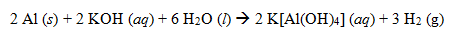Since the hydrogen gas is separated from the K[Al(OH)4] ion, the next step is to convert this into alum by reacting it with sulfuric acid, H2SO4.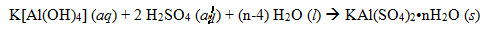Since the hydrogen gas is separated from the K[Al(OH)4] ion, the next step is to convert this into alum by reacting it with sulfuric acid, H2SO4.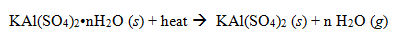There are three different qualitative tests that are performed to prove the presence of K+, Al3+, and SO­42- ions in the double salt: a flame test for potassium, a red lake test for aluminum, and a precipitation test for sulfate. Lastly, the experimental yield of alum product that was measured is used to calculate the percent yield of the synthesis. The percent yield can be calculated using the following equation: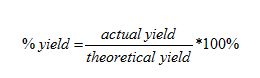Aluminum is the limiting reagent in this experiment, so that the theoretical moles of alum product will be equal to the moles of Al consumed. Once the empirical formula for alum has been determined, the theoretical yield can be determined using the molar mass and the moles of Al reacted. The actual yield is the number of grams of alum product received.

Experimental Method

Week 1:

Week 2:

A tared blue weighing boat was placed onto the balance and the balanced was zeroed. The solid was scraped off of the watch glass and placed into the weighing boat. The exact mass of the crystals was recorded. Two crucibles were cleaned with 2 mL of ammonia solution and dried with a paper towel. An iron ring was attached to a ring stand and a clay triangle was placed on top of the iron ring. One of the crucibles was placed into the clay triangle. A Bunsen burner was position beneath the clay triangle so that the hottest part of the flame was in contact with the crucible. The crucible was heated for 5 minutes until it was red hot. The same was done for the other crucible. The crucibles were cooled on wired gauze for 5 minutes. After they were cooled, each crucible was weighed on an analytical balance and the mass of each was recorded. The balance was zeroed and about 0.8 g of alum sample was placed into each crucible. The exact mass of the alum sample added was recorded. Then the exact mass of each crucible with the alum sample was recorded. The crucibles were placed in a small beaker and placed in the oven at 110 °C for one hour. During this hour, the qualitative tests were completed. The first was the flame test for potassium. About 1 mL of concentrated HCl was measured in a small beaker. The loop end of clean nichrome wire was dipped into the concentrated HCl and then dipped into a small sample of the leftover alum. The Bunsen burner was lit, and the loop was placed in the hottest part of the flame, which was the tip of the blue inner cone, while looking through a blue cobalt filter. The second test was the precipitation test for sulfate. A small amount of alum was dissolved in a test tube in minimum amount of water. Using a dropper, 0.20 M barium nitrate solution was added. The test tube’s contents were discarded in the provided container. The final qualitative test was the red lake test for aluminum. A small amount of alum was dissolved in a test tube using a minimum amount of 1M HNO. Several drops of aluminon indicator were also added. The mixture was shaken to mix the contents well and 6 M NH3 (aq) was added dropwise until the solution was basic. The beaker was removed from the oven and cooled for 5 minutes. Tongs were used to handle each crucible. Each crucible was then weighed with its contents on the analytical balance and the mass was recorded. Each crucible was placed back into the beaker and into the oven for another 30 minutes. The beaker was removed and cooled for 5 minutes. Each crucible was weighted and the mass of each was recorded.

Results
The masses of each empty crucible, each crucible with the contents after each heating, the mass of dehydrated alum, the mass of water lost, and the percent of H­2O in alum by mass are listed in Table 1. The average percent of H2O by mass was ascertained to be 29.46%. Using the mole ratio of moles of H2O to moles of KAl(SO4)2, the value of n in the empirical formula of alum was determined to be 6, therefore making the empirical formula of this sample of alum to be KAl(SO4)2•6H2O. The molar mass using this empirical formula of alum was calculated to be 366.3 g/mol. The overall set of balanced equations for the synthesis of alum was determined to be: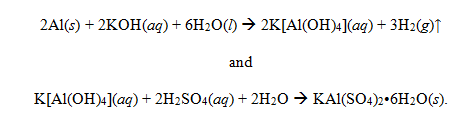The theoretical yield of alum was determined to be 6.908 g. The actual yield was 3.676 g. Therefore, there was a 53.21% yield. The actual empirical formula of alum is KAl(SO4)2•12H2O. The percent of H2O from this formula is 45.57%1. Therefore, there was a 35.35% error in this experiment. There was also a positive test for each of the qualitative test: a lavender flame was present to the presence of potassium, a white precipitate of BaSO4 was formed to indicate the presence of sulfate, and a red precipitate was formed to indicate the presence of aluminum.

Table 1: The masses of each empty crucible, each crucible with the contents after each heating, the mass of dehydrated alum, the mass of water lost, and the percent of H­2O in alum by mass

 Crucible 1 Crucible 2 Mass (in g) of empty crucible 11.8023 20.9243 Mass (in g) of crucible/contents after 1st heating 12. 3672 21.4978 Mass (in g) of crucible/contents after 2nd heating 12.3666 21.4890 Mass (in g) of dehydrated alum 0.5643 0.5647 Mass (in g) of H2O lost 0.2359 0.2356 % H2O in alum by mass 29.48% 29.44%

Discussion

The mass of H2O lost in crucible 1 and crucible 2 was 0.2359 g and 0.2356 g respectively. Therefore, for crucible 1 and crucible 2, there was 29.48 % H2O and 29.44 % H2O in each respective sample of alum. The average of these percentages is 29.46% H2O. Therefore, one can assume that in a 100 g sample of alum, there would be 29.46 g of H2O. Dividing this value by the molar mass of H2O calculates to 1.636 mol H2O. If there is 29.46 g of H2O present in a 100g sample of alum, then there is 70.54 g of KAl(SO42. Dividing this value by the molar mass of KAl(SO42 calculates to 0.2732 mol KAl(SO42. Dividing 1.636 mol H2O by 0.2732 mol KAl(SO42 calculates to 5.988, which rounded to the nearest integer is 6. Therefore, in this sample of alum synthesized, there are 6 waters of hydration (n is equal to 6 in the empirical formula). The actual value of n for alum is 12. That means that the actual percentage of H2O in alum is supposed to be 45.57%. Therefore, in this experiment, there was a 35.35% error. Plausible sources of error include the fact that the Büchner funnel fell when the alum was being transferred to the watch glass, resulting in a loss of actual yield; the crucibles were probably not heated for the entire one hour during the first heating or for the entire 30 minutes during the second heating, resulting in an incomplete dehydration; or additional mass added to the actual yield from rust or other substances left on the spatula.

References

1Intensity(I/I. (n.d.). Alum-(K) Mineral Data. Mineralogy Database. Retrieved September 26, 2012, from http://webmineral.com/data/Alum-(K).shtml#.UGJzb7JmSNI

• Share